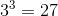# SAT II Math I : Ratios and Proportions

## Example Questions

### Example Question #15 : How To Find Percentage From A Fraction

Convert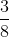into a percentage.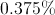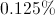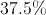None of the other answers are correct.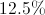Explanation:

Set up a proportion: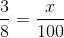Cross-multiply: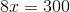Isolateby dividing by 8: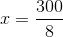Finally, simplify: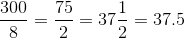The fraction is therefore equivalent to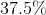.

### Example Question #1 : Ratios And Proportions

Convert the following fraction into a percent.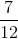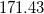%

.63 %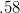%

63.26 %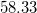%%

Explanation:

Set up a proportion: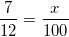Cross multiply: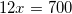Isolateby dividing both sides by 12: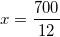Simplify: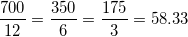(Hint: If you are unable to use long division to solve the fraction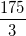, take a look at your answer choices and see if you can narrow down your choices. You know that value is going to be greater than one, since 175 is greater than 3. You also know that dividing any number by 3 is going to result in a decimal of .33, .66, or no decimal at all. With these two pieces of information, you should be able to isolate the correct answer without actually solving for it like I did in the last step.)

### Example Question #1 : Ratios And Proportions

Solve for: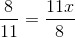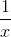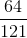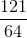Explanation:

To solve this proportion, cross multiply.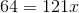From here to solve, divide both sides by 121.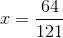### Example Question #3 : Ratios And Proportions

If for everyinch on a map it representsmiles, how many inches will be needed for a distance of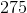miles?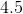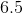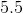Explanation:

The ratio of inches to miles is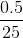so you can set up a proportion of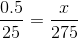.

You then cross multiply to get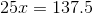.

Then you solve for the variable by dividing bywhich gives us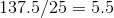.

### Example Question #4 : Ratios And Proportions

In a geometric sequence the ratio of the first term to the third term is 9:1. What is the ratio of the second term to the fifth term?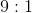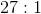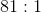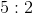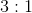Explanation:

Recall that in a geometric sequence there is a constant ratio between any pair of consecutive terms: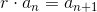If the ratio of the first term to the third term is 9:1 then the ratio of two consecutive terms is a number that when multiplied by itself is 9: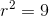Therefore the common ratio for this sequence is 3.

There are three terms between term 2 and 5 so the common ratio will be applied to the second term three times to get the fifth term: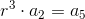Since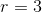for this sequence